If you’re a fan of brain teasers and puzzles, you might have come across the following set of equations:

Cat + Cat + Cat = 60
Cat + 3 Eggs + 3 Eggs = 26
3 Eggs + 6 Bananas + 6 Bananas = 15
Cat + 2 Eggs x 4 Bananas = 28

At first glance, these equations may seem simple, but they are designed to challenge your ability to think outside the box and solve problems creatively.

Let’s break it down step by step. In the first equation, we have three cats adding up to 60. If we divide 60 by 3, we get 20. Therefore, we can conclude that each cat is equal to 20.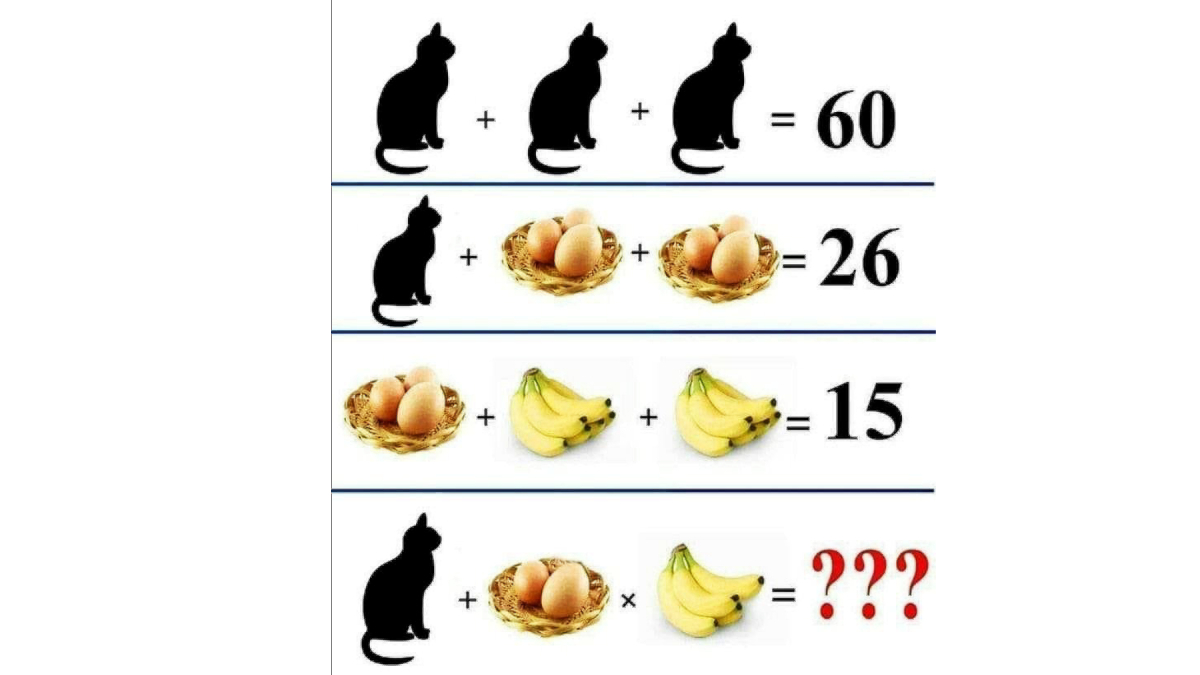Moving on to the second equation, we have two sets of three eggs and a cat, adding up to 26. Since we know that a cat is equal to 20, we can subtract 20 from 26, leaving us with 6. That means the total value of six eggs is equal to 6. To find the value of one egg, we divide 6 by 6, which gives us 1.

Now, let’s look at the third equation. We have two sets of six bananas and one set of three eggs adding up to 15. Since we know that one egg is equal to 1, we can subtract 3 from 15, which leaves us with 12. That means the total value of 12 bananas is equal to 12. To find the value of one banana, we divide 12 by 12, which gives us 1.

Finally, we come to the last equation, which is perhaps the trickiest of them all. We have one cat, two sets of eggs (each set consisting of three eggs), and four bananas. We know that one cat is equal to 20, and one egg is equal to 1. To find the value of four bananas, we multiply 4 by 1, which gives us 4.

Now we have all the information we need to solve the equation. We can substitute the values we have found for each item:

Cat + 2 Eggs x 4 Bananas = 20 + 2(1) x 4(1) = 28

So the answer to the puzzle is 28.

In conclusion, solving puzzles like this requires a bit of creativity and logic. It’s essential to break down the problem into smaller parts, identify patterns and connections, and use deduction to find the solution. Hopefully, this article has shed some light on how to approach this particular puzzle and perhaps even inspired you to take on more brain teasers in the future.

## Related News#### Challenge to science: Treasure full of gold and pearls guarded by snake god found [PhotoFeature]#### For the first time in the world : a woman was conceived by a robot, twins were born#### Indonesian mermaids breast : इंडोनेशिया में जलपरियों के स्तन क्यों ढका जा रहा है ?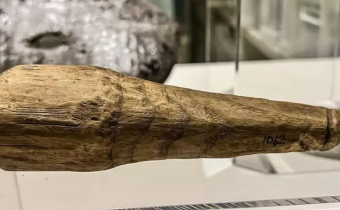#### A 2,000-year-old ‘sex toy’ is found : you’ll be shocked to hear what the searchers are saying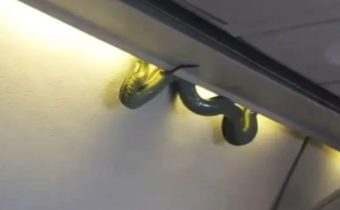#### When the snake suddenly started running inside the flying plane !#### Manchineel: दुनिया का सबसे ख़तरनाक पेड़, फल का एक टुकड़ा खाने से ही हो सकती है मौत !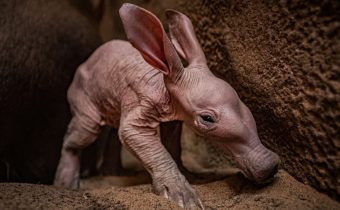#### 90 साल बाद पैदा हुआ ये दुर्लभ जानवर, बड़े कान-बिना बाल के Harry Potter के एक किरदार जैसा दिखता है#### फ्लाइट में सेक्स Sex in flight : पत्नी के जन्मदिन पर पति का सरप्राइज गिफ्ट#### ‘मातम में सेक्स बेच रहे’, अंतिम संस्कार के विज्ञापन में नग्न मॉडल को लेकर हो रहे हंगामे#### दोस्त ने कहा, “अरे आपकी बेटी एडल्ट साइट OnlyFans पर है, तो पापा के रिएक्शन से दुनिया हैरान है” !#### एक आदमी अपनी पत्नी को तलाक देने की कारण जानकर दुनिया हैरान !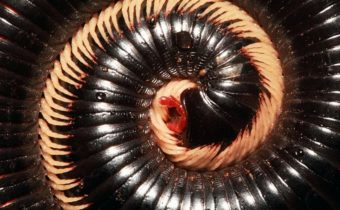#### 1,306 पैरों वाला दुनिया का पहला अनोखा जीव ऑस्ट्रेलिया में पाया !#### ‘मुझे पैदा क्यों किया’, जन्म देने के आरोप में लड़की ने मां के डॉक्टर पर किया केस, मिले करोड़ों रुपए#### बैग में अपने हृदय से घूम रही है यह महिला : मिलना चाहती है ? the woman who carries her heart in a bag